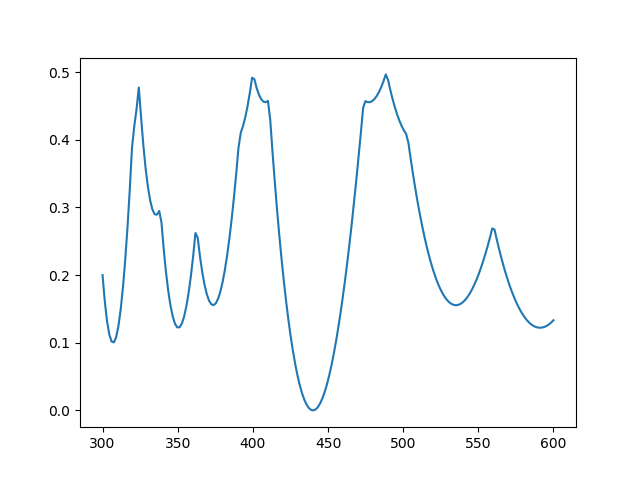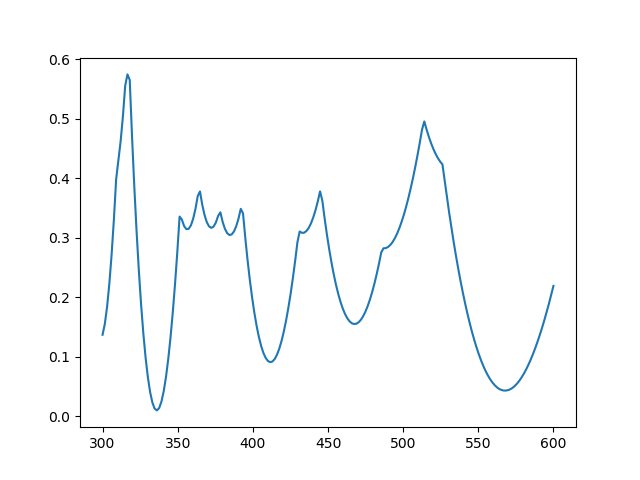# Determining fundamental frequency

My daughter had a homework problem the other day that gave the frequencies of several Fourier components and asked her to find the fundamental frequency. The numbers were nice enough that brute force worked, and I’m sure that’s what students were expected to do. But this could easily be a much more sophisticated problem.

If the frequencies are all integers and exact multiples of a fundamental frequency, you can simply take the greatest common divisor of the frequencies. If you’re told the frequencies are 1760, 2200, and 3080, then the fundamental frequency is apparently 440 since that’s the greatest common divisor.

But what if the data are a little different? Say the highest pitch is 3081. Surely 440 should still be considered the fundamental frequency, even though now the greatest common divisor of the frequencies would be 1 Hz. What if the highest frequency was 3078 + π? Surely the fundamental frequency is still 440 for practical purposes.

And what might these practical purposes be? One purpose might be pitch detection. When several frequencies are combined that are small integer multiples of a fundamental frequency, we perceive the combination as having pitch given by that fundamental.

For something like a guitar string, the frequency components are close to small integer multiples of a fundamental frequency. But for something like a church bell, the frequencies don’t line up so neatly, though there’s still a clearly perceived pitch. For something like a metal mixing bowl, it may be difficulty to predict what pitch a person will hear when something strikes the bowl.

One complication we haven’t addressed yet is that the fundamental frequency will not be unique without some constraint. In the example above, the frequencies were all multiples of 440, but they’re also all multiples of 440/n for every positive integer n. We might get around this by specifying some lower bound on the fundamental frequency. Or we could say that all other things being equal, we want the largest candidate for the fundamental frequency.

We could formulate the problem of finding the fundamental frequency as an optimization problem. For example, we could form a mixed integer program. Suppose we have three frequencies f1, f2, and f3. We could find a fundamental frequency f and integers n1, n2, and n3 that minimize

(f1n1 f)² + (f2n2 f)² + (f3n3 f

subject to a lower bound on f.

We can eliminate the explicit dependence on the integer coefficients by minimizing

(f1/f – [f1/f])² + (f2/f – [f2/f])² + (f3/f – [f3/f])² .

where [x] denotes nearest integer to x. The first formulation has a more common form. The latter has a more complicated objective function, but it’s only a function of one variable.

Here’s what the latter looks like for frequencies 1760, 2200, and 3080.Clearly there’s a minimum at 440 Hz.

Here’s the same plot with 10% random noise  added to each frequency: 1701, 2368, and 3339.Now there’s a minimum near 336, but the local minimum at 566 is nearly as good.

## Related posts

 There are a couple reasons you might want to solve a problem like this. Maybe your frequencies really are integer multiples of a fundamental frequency, but there is measurement error. Another is that the frequencies are not exactly multiples of a fundamental, as when striking a bell or a mixing bowl. How might you formulate the two cases differently?

## 3 thoughts on “Determining fundamental frequency”

1. Saurish Chakrabarty

It would be interesting to compare the first plot with an average over all possible realizations of the plot with 10% noise. Are they identical? If not, at what value of the noise do they stop being identical?

In other words, does a large collection of defective church bells produce the same qualitative sound as the same number of ideal church bells? How defective can these church bells be?

2. Hi John. Do you have any references for the construction and solution of the two optimizations you suggest?
Thanks

3. I made up the construction in the blog post. It’s probably not original.

If you want to do pitch detection, then you have a much more difficult problem than solving a mathematically clean optimization problem. What do people perceive as the fundamental? We know that doesn’t have a unique answer. You’d have to settle for saying what most people perceive as the fundamental under these circumstances, or saying something about the range of perceptions and how common each is etc.

If you have clean, approximately harmonic frequency ratios then there’s broad agreement regarding the pitch. But if the ratios are much messier, as they often are with bells, there’s less agreement. It would probably be hard to say, for example, what pitch people heard when the Liberty Bell rang.